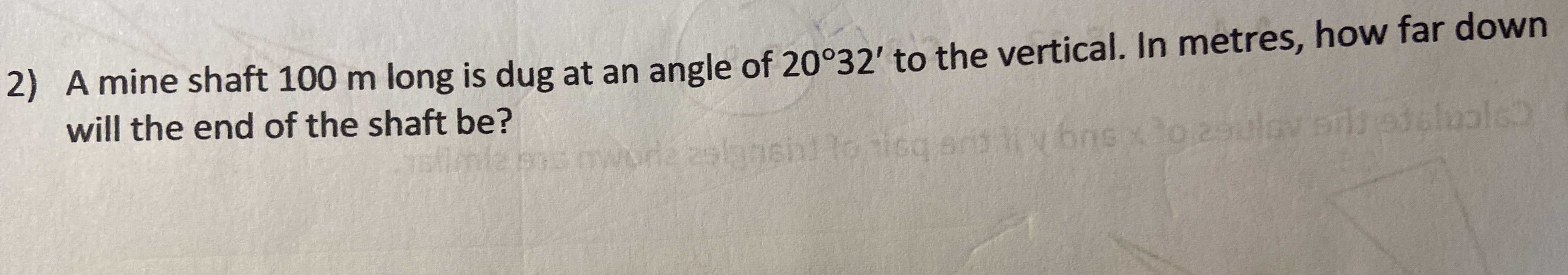### Still have math questions?A mine shaft $$100 m$$ long is dug at an angle of $$20 ^ { \circ } 32 ^ { \prime }$$ to the vertical. In metres, how far down will the end of the shaft be?
100×cos(20$$°$$32')=93.6 m